## Wednesday, July 10, 2013

### 6Tempdb contention and solution

I want to see how much difference by adding multiple tempdb data files and cache the temp table plan could make.

## setup test environment

`USE [master]`
`GO`
`ALTER DATABASE [tempdb] MODIFY FILE ( NAME = N'tempdev', SIZE = 4096Mb);`
`ALTER DATABASE [tempdb] MODIFY FILE ( NAME = N'templog', SIZE = 1024Mb)`
`GO`
`-- bounce sql server`
`use tempdb;`
`checkpoint;`

## No caching/MIX extend/single data file

"C:\Program Files\Microsoft Corporation\RMLUtils\ostress" -E -itempbatch.sql -S. -n100 -r50 –q

The test took about 2 and half minutes to complete.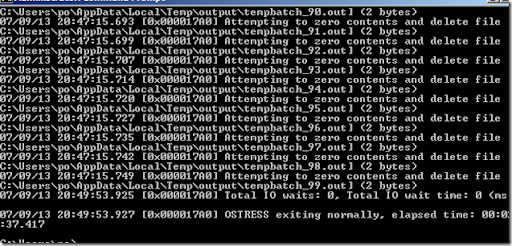`create table #x (c1 int,c2 char(7000) not null)`
`CREATE UNIQUE CLUSTERED INDEX idx1 ON #x ( c1 ) ;`
`SET nocount ON ;`
`    DECLARE @i INT = 0 ;`
`    WHILE ( @i < 10 )`
`        BEGIN`
`           insert into #x values (@i,'sunny')`
`           SET  @i += 1 ;`
`        END ;`
`select COUNT(*) from #x;`
`drop table #x`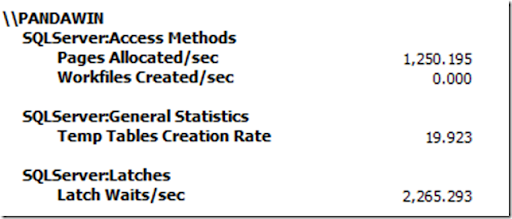Below query check to see the tempdb contention is happening at PFS page with PAGELATCH wait.

`select * from sys.dm_os_waiting_tasks `
` --where resource_description like '2%'`
`where resource_description ='2:1:1' `
`or resource_description ='2:1:2' `
`or resource_description ='2:1:3'`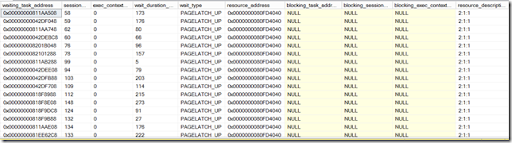`dbcc traceon(1106, -1)`
` `
`declare @ts_now bigint`
`select @ts_now=cpu_ticks/(cpu_ticks/ms_ticks) from sys.dm_os_sys_info`
`select record_id,`
`dateadd(ms, -1 * (@ts_now - [timestamp]), GetDate()) as EventTime,`
`CASE `
`    WHEN event = 0 THEN 'Allocation Cache Init'`
`    WHEN event = 1 THEN 'Allocation Cache Add Entry'`
`    WHEN event = 2 THEN 'Allocation Cache RMV Entry'`
`    WHEN event = 3 THEN 'Allocation Cache Reinit'`
`    WHEN event = 4 THEN 'Allocation Cache Free'`
`    WHEN event = 5 THEN 'Truncate Allocation Unit'`
`    WHEN event = 10 THEN 'PFS Alloc Page'`
`    WHEN event = 11 THEN 'PFS Dealloc Page'`
`    WHEN event = 20 THEN 'IAM Set Bit'`
`    WHEN event = 21 THEN 'IAM Clear Bit'`
`    WHEN event = 22 THEN 'GAM Set Bit'`
`    WHEN event = 23 THEN 'GAM Clear Bit'`
`    WHEN event = 24 THEN 'SGAM Set Bit'`
`    WHEN event = 25 THEN 'SGAM Clear Bit'`
`    WHEN event = 26 THEN 'SGAM Set Bit NX'`
`    WHEN event = 27 THEN 'SGAM Clear Bit NX'`
`    WHEN event = 28 THEN 'GAM_ZAP_EXTENT'`
`    WHEN event = 40 THEN 'FORMAT IAM PAGE'`
`    WHEN event = 41 THEN 'FORMAT PAGE'`
`    WHEN event = 42 THEN 'REASSIGN IAM PAGE'`
`    WHEN event = 50 THEN 'Worktable Cache Add IAM'`
`    WHEN event = 51 THEN 'Worktable Cache Add Page'`
`    WHEN event = 52 THEN 'Worktable Cache RMV IAM'`
`    WHEN event = 53 THEN 'Worktable Cache RMV Page'`
`    WHEN event = 61 THEN 'IAM Cache Destroy'`
`    WHEN event = 62 THEN 'IAM Cache Add Page'`
`    WHEN event = 63 THEN 'IAM Cache Refresh Requested'`
`    ELSE 'Unknown Event'`
`END,`
`session_id,`
`page_id,`
`allocation_unit_id`
`from`
`(`
`select `
`xml_record.value('(./Record/@id)', 'int') as record_id,`
`xml_record.value('(./Record/ALLOC/Event)', 'int') as event,`
`xml_record.value('(./Record/ALLOC/SpId)', 'int') as session_id,`
`xml_record.value('(./Record/ALLOC/PageId)', 'varchar(100)') as page_id,`
`xml_record.value('(./Record/ALLOC/AuId)', 'varchar(100)') as allocation_unit_id,`
`timestamp`
`from `
`    (`
`    select timestamp, convert(xml, record) as xml_record`
`    from sys.dm_os_ring_buffers`
`    where ring_buffer_type = N'RING_BUFFER_ALLOC_TRACE'`
`    ) as the_record`
`) as ring_buffer_record`
`-- where ring_buffer_record.event = 10`
`--order by EventTime`
`order by record_id`
`go`
` `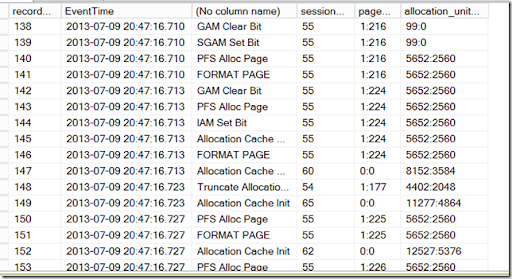## CACHE TEMP table/Multiple tempdb data files

Before re run the test:

• Bounce sql server.
• Truncate the tempdb log by issuing hard checkpoint
`ALTER DATABASE [tempdb] MODIFY FILE ( NAME = N'tempdev', SIZE = 1024MB);`
`GO`
`ALTER DATABASE [tempdb] ADD FILE ( NAME = N'tempdev2', FILENAME = N'C:\Program Files\Microsoft SQL Server\MSSQL10_50.MSSQLSERVER\MSSQL\DATA\tempdev2.ndf' , SIZE = 1024MB , FILEGROWTH = 10%)`
`GO`
`ALTER DATABASE [tempdb] ADD FILE ( NAME = N'tempdev3', FILENAME = N'C:\Program Files\Microsoft SQL Server\MSSQL10_50.MSSQLSERVER\MSSQL\DATA\tempdev3.ndf' , SIZE = 1024MB , FILEGROWTH = 10%)`
`GO`
`ALTER DATABASE [tempdb] ADD FILE ( NAME = N'tempdev4', FILENAME = N'C:\Program Files\Microsoft SQL Server\MSSQL10_50.MSSQLSERVER\MSSQL\DATA\tempdev4.ndf' , SIZE = 1024MB , FILEGROWTH = 10%)`
`GO`

The SQL server can only cache the temp table with the the query plan, therefore we need to change our dynamic sql to a store procedure.

`use master`
`go`
`drop proc sp_summer1`
`go`
`create proc sp_summer1`
`as`
`create table #x (c1 int unique clustered,c2 char(7000) not null)`
`SET nocount ON ;`
`    DECLARE @i INT = 0 ;`
`    WHILE ( @i < 10 )`
`        BEGIN`
`           insert into #x values (@i,'sunny')`
`           SET  @i += 1 ;`
`        END ;`
`select COUNT(*) from #x;`
`drop table #x`
`go`
`select object_id('sp_summer1')`
`go`

Here it the ostress command I run

ostress.exe -S. -Q"exec master.dbo.sp_summer1" -n100 -r50

It tooks 14 secs to complete the testing.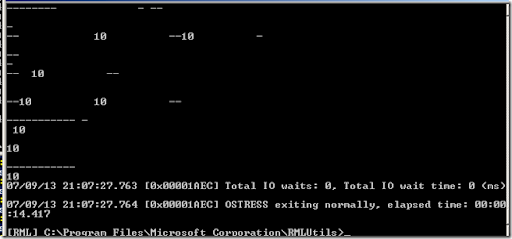We still get PAGELATCH contention but it is spread out multiple files.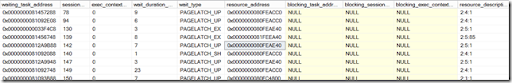os ring buffer also show the page allocation across multiple files.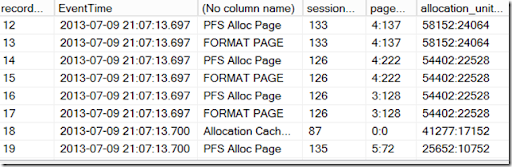Temp table creation rate is 0 .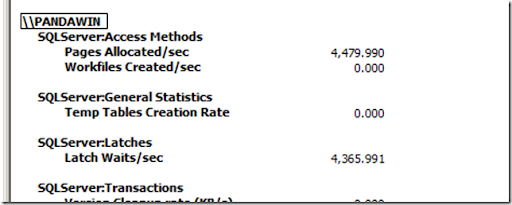log shows the table caching

`select name,object_id,type,type_desc from sys.objects where name like '#%'`
`select Operation, Context, [Transaction ID], [Log Record Length], AllocUnitName, `
`[Transaction Name], [RowLog Contents 0], [RowLog Contents 1], `
`[RowLog Contents 2], [RowLog Contents 3],[Number of Locks],[Lock Information]`
`from sys.fn_dblog(null, null);`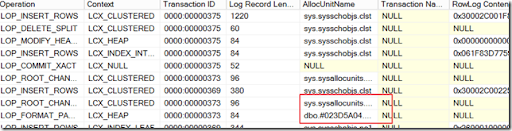## conclusion

By adding multiple tempdb files and cache the temp table plan. we dramatically reduce the query exaction time .

Before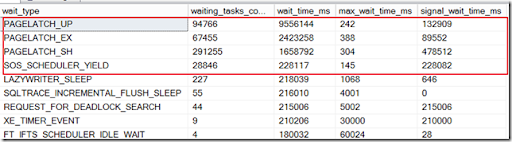After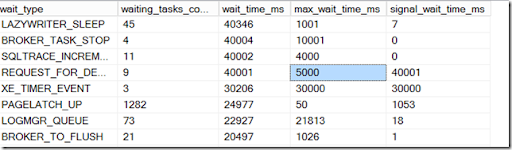Further optimize

If you see the contention on the SGAM page, we can turn on the trace flag 1118 so sql server would use uniform extend instead of mix extend.  However, in this particular case, it does not make much difference.

`dbcc traceon(1118,-1)`

1.Thanks for sharing this, I actually appreciate you taking the time to share with everybody.
Best Data Science Course In Hyderabad

2.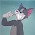fake moncler jackets Shop the Latest Men's fake moncler men jackets Collections from Moncler.

3.4.i have to thank you for the time i spent on this especially great reading !! i really liked each part and also bookmarked you for new information on your site...

5.6.